Instant Maths Help:

# Edexcel A Level

## Solving Trig Equations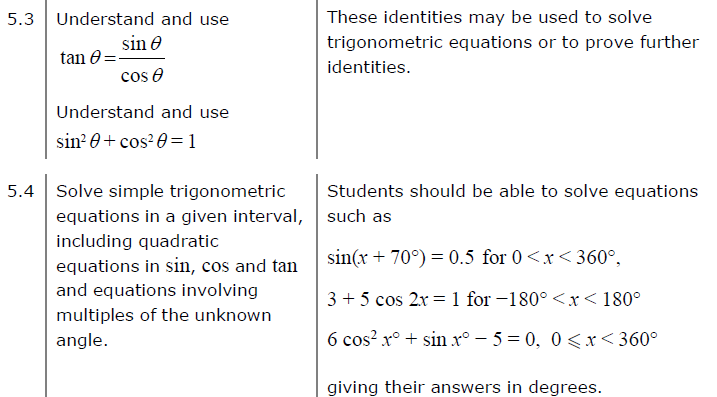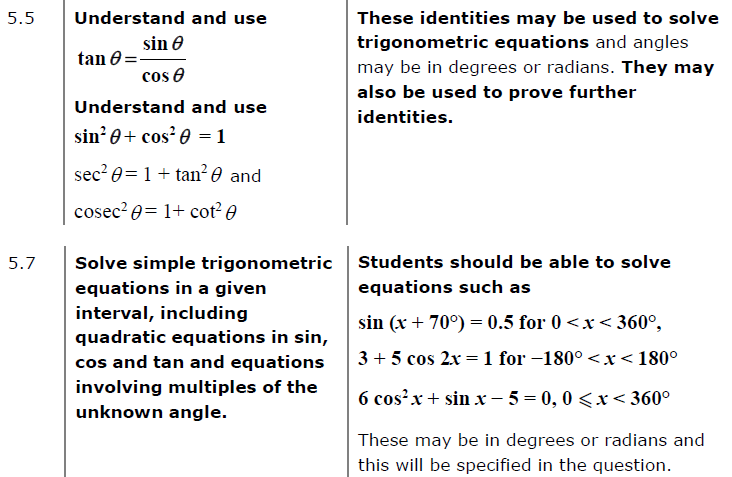##### Edexcel 8MA0/01 Jun 2023 AS Exam Q. 12 bc :   6 marks in 7:12 min.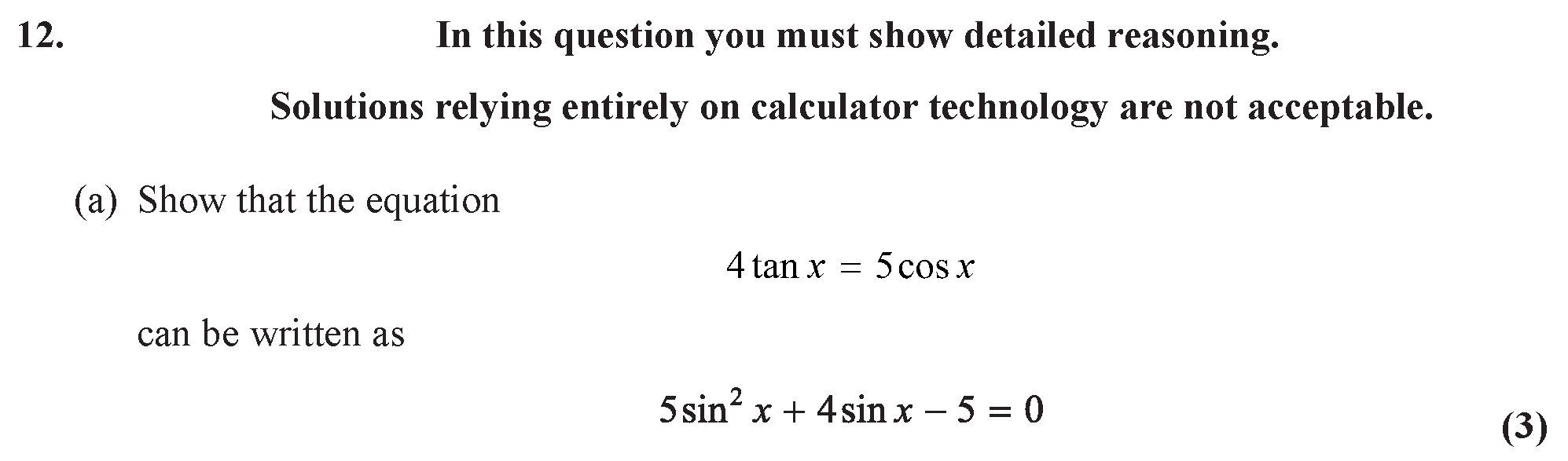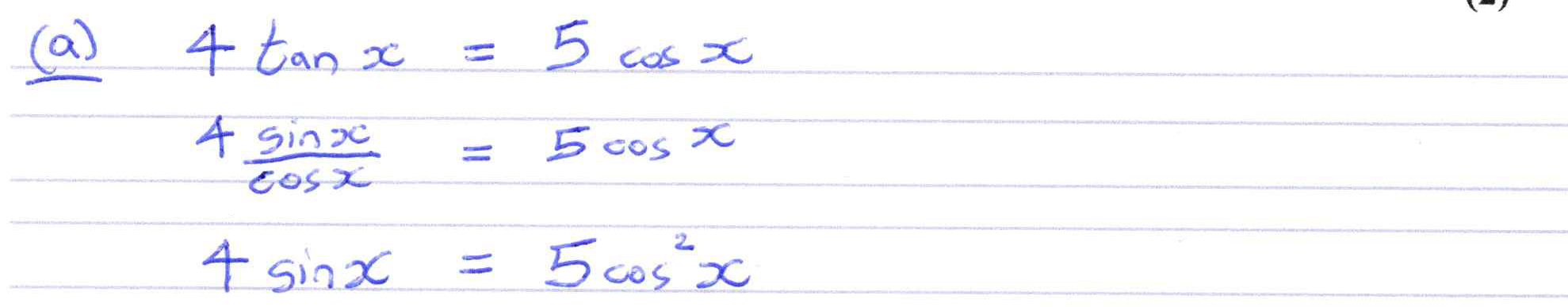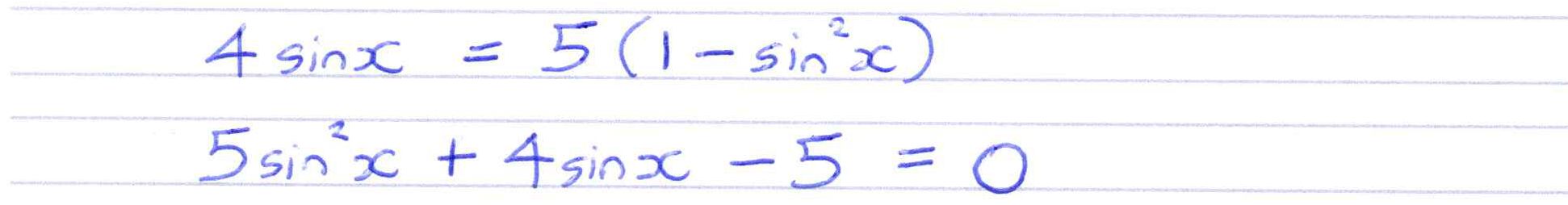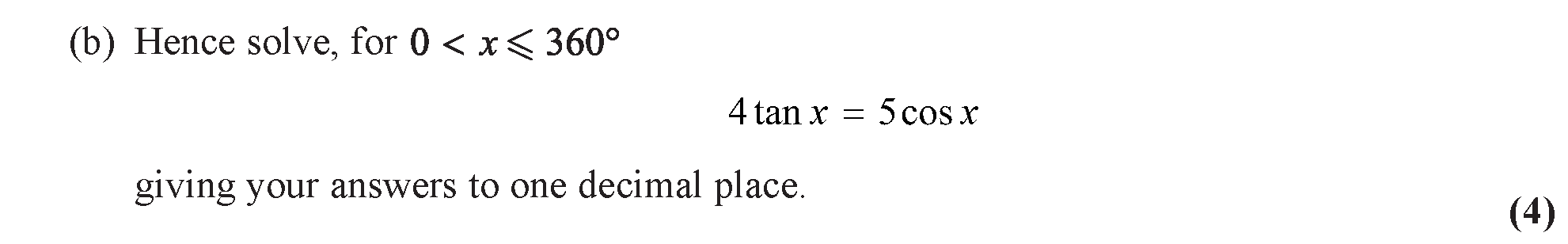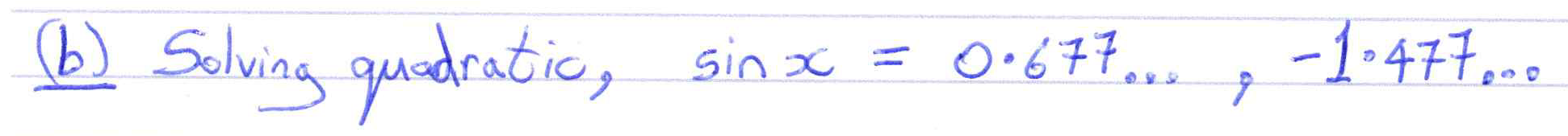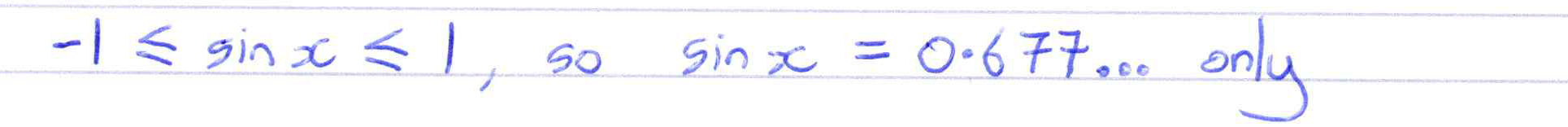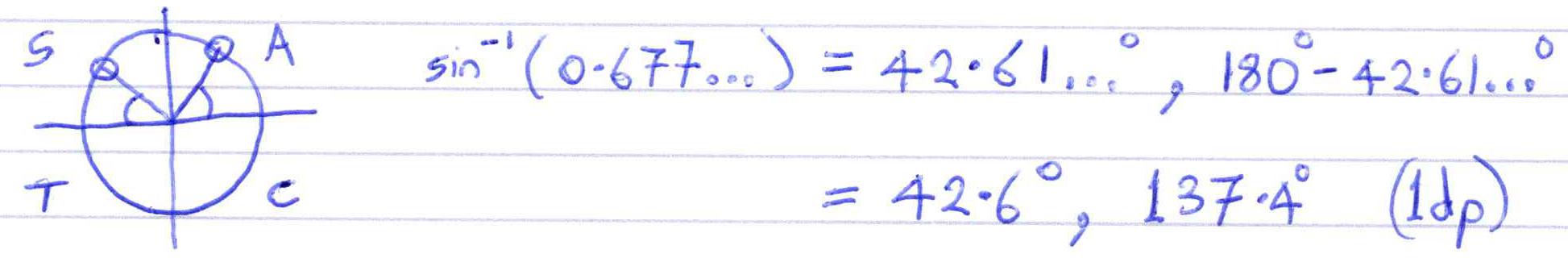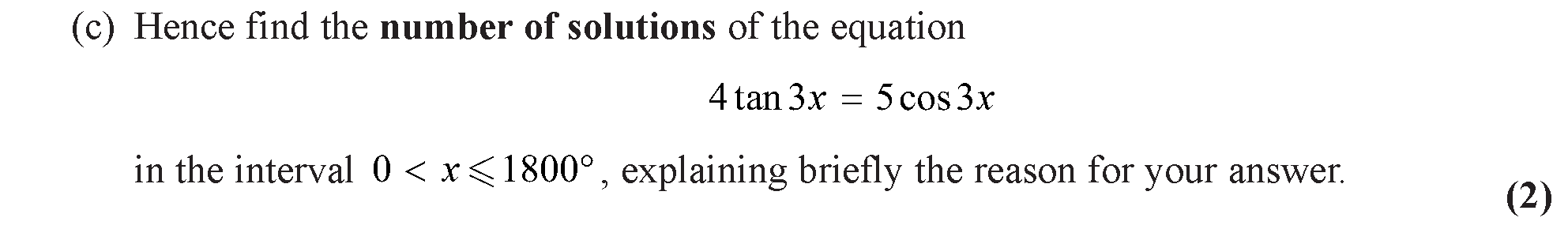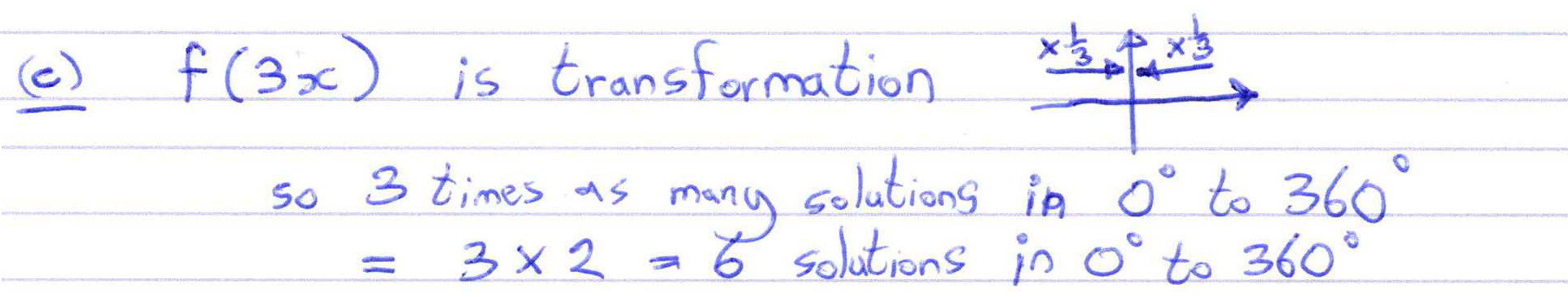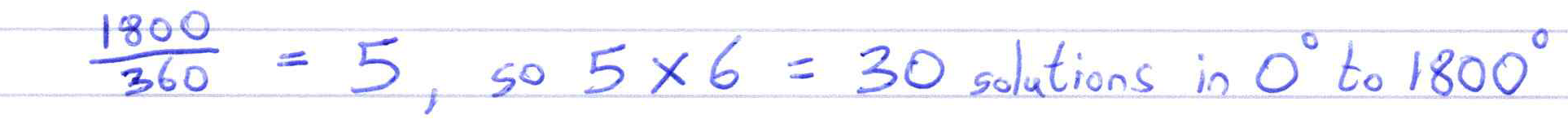##### Edexcel 9MA0/02 Jun 2023 A2 Exam Q. 14 b :   4 marks in 4:48 min.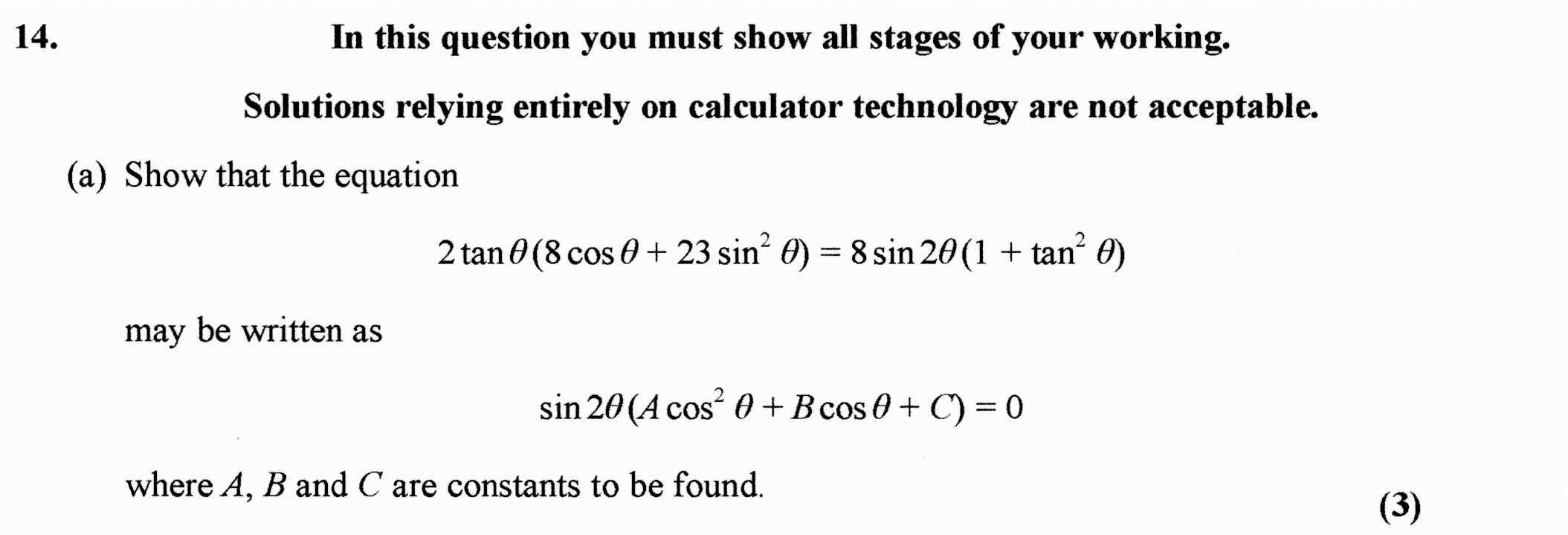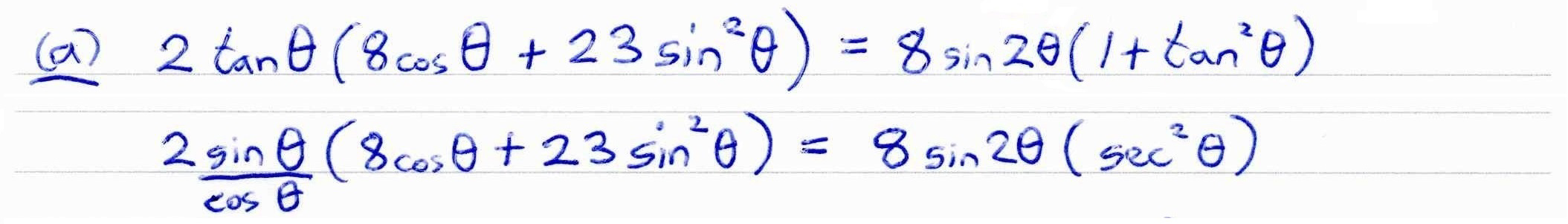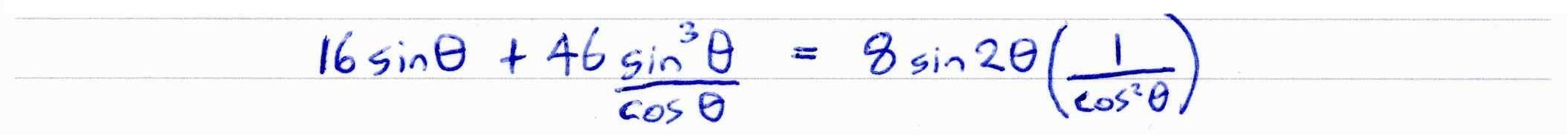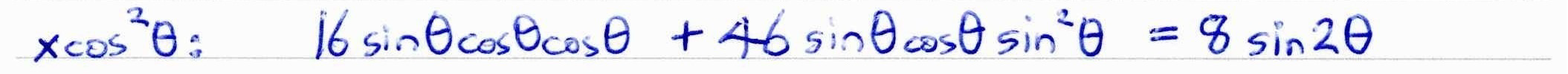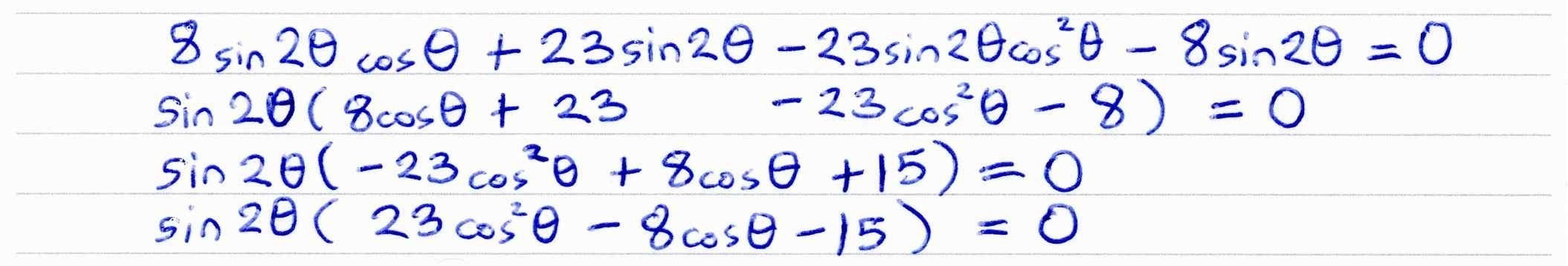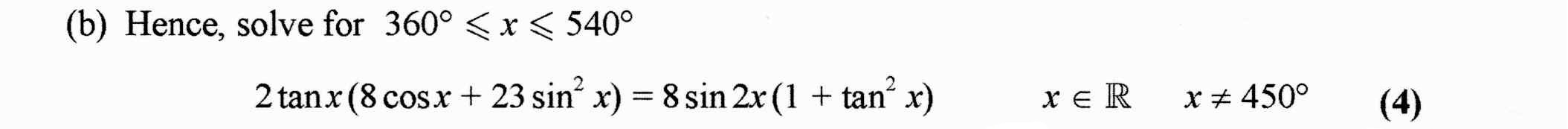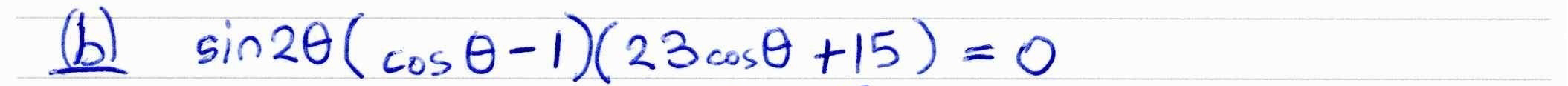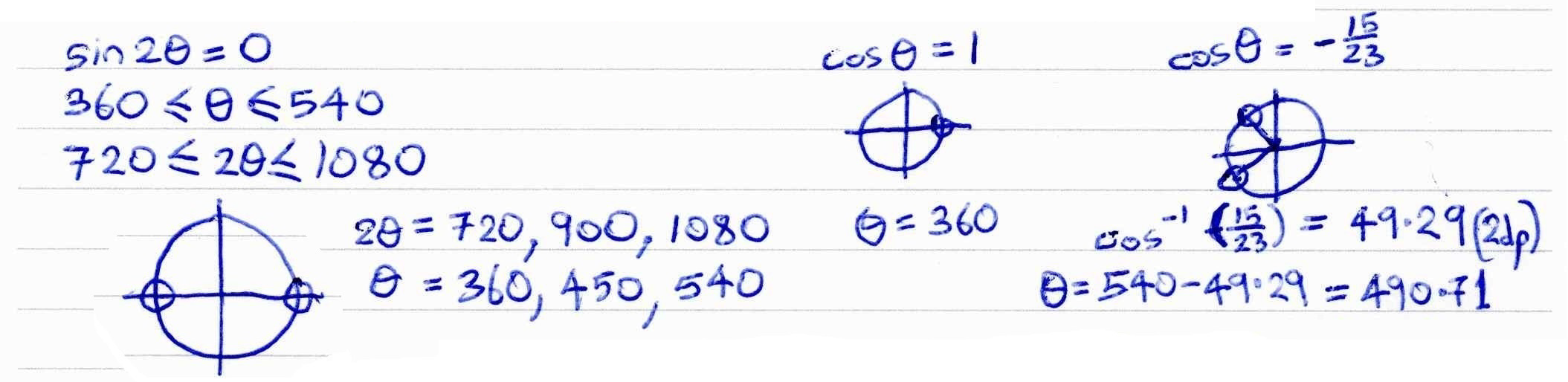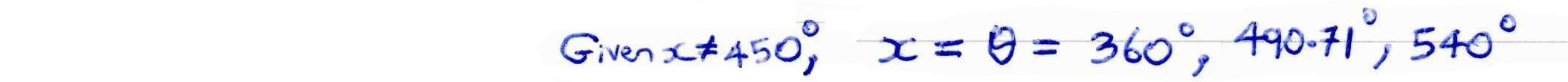##### Check solutions to equations numerically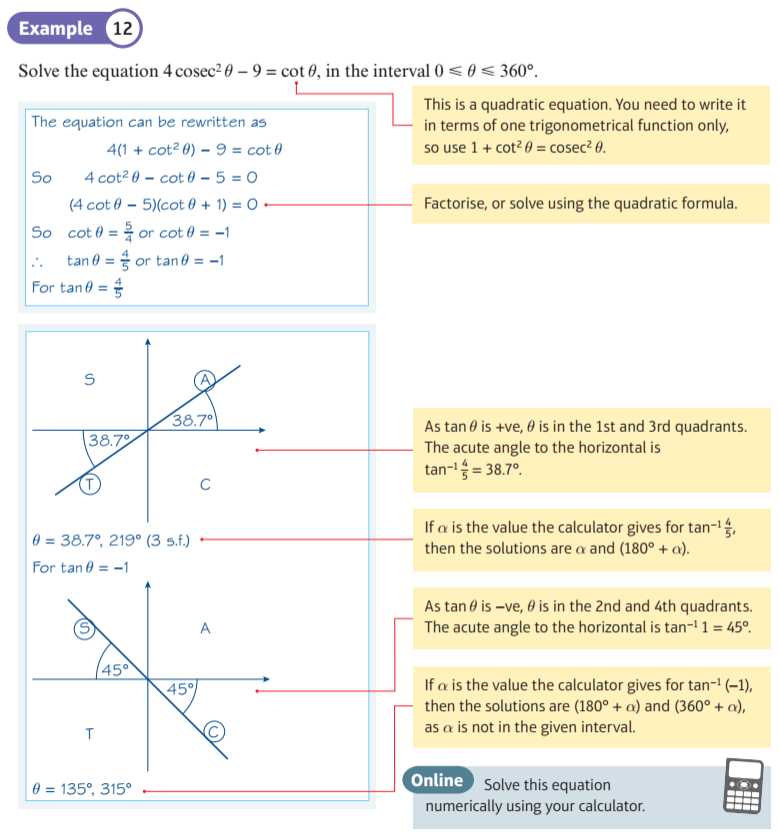##### Find Derived Identity 1
Two other identities can be derived from the identity ${\sin ^2}(x) + {\cos ^2}(x) \equiv 1$. This display allows you to view one of them.

Adjust the sliders so the green and red curves coincide. You will see the identity.
##### Find Derived Identity 2
Two other identities can be derived from the identity ${\sin ^2}(x) + {\cos ^2}(x) \equiv 1$. This display allows you to view one of them.

Adjust the sliders so the green and red curves coincide. You will see the identity.